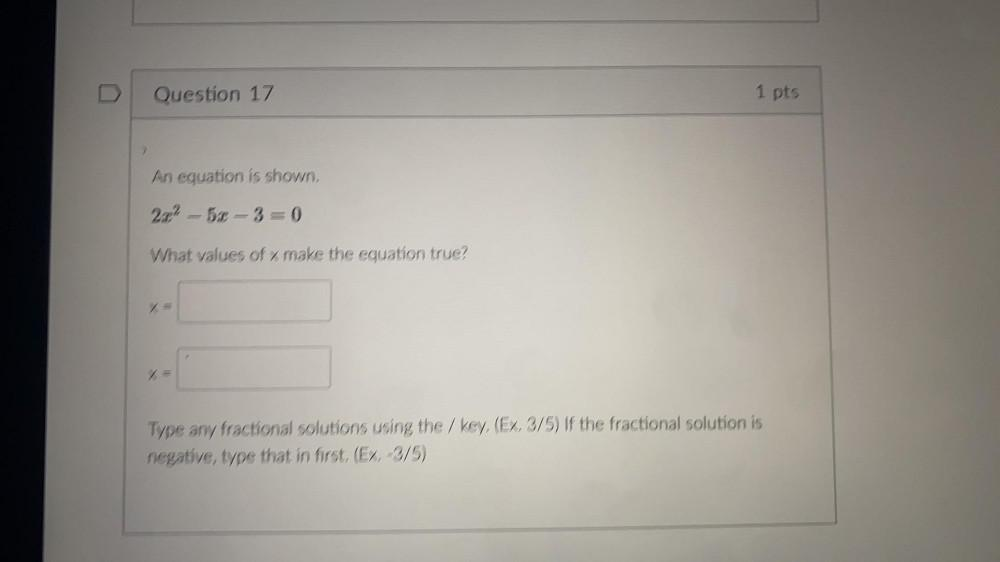Question:

# An equation is shown. 2x²5x-3=0 What values of x make theAn equation is shown. 2x²5x-3=0 What values of x make the equation true? x= y= Type any fractional solutions using the / key. (Ex. 3/5) If the fractional solution is negative, type that in first. (Ex. -3/5)